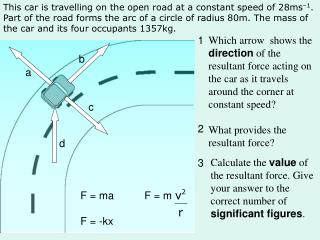DownloadDownload PresentationF = ma F = m F = -kx

# F = ma F = m F = -kx

Download Presentation## F = ma F = m F = -kx

- - - - - - - - - - - - - - - - - - - - - - - - - - - E N D - - - - - - - - - - - - - - - - - - - - - - - - - - -
##### Presentation Transcript

1. This car is travelling on the open road at a constant speed of 28ms–1. Part of the road forms the arc of a circle of radius 80m. The mass of the car and its four occupants 1357kg. 1 Which arrow shows the direction of the resultant force acting on the car as it travels around the corner at constant speed? What provides the resultant force? 2 Calculate the value of the resultant force. Give your answer to the correct number of significant figures. 3 F = ma F = m F = -kx b a c d

2. This car is travelling on the open road at a constant speed of 28ms–1. Part of the road forms the arc of a circle of radius 82m. The mass of the car and its four occupants 1357kg. C Friction between the tyres and the road. F = m b a c d F = 1375 x F = 12974 F= 13000 N (2 sig fig) F = ma F = m F = -kx